# 不同质量图在卡尔曼滤波相位解缠中应用探讨The Study on Application of Different Quality Maps in Kalman Filter Phase Unwrapping

DOI: 10.12677/GST.2019.72011, PDF, HTML, XML, 下载: 576  浏览: 1,285

Abstract: The Kalman Filter transforms the phase unwrapping problem into state estimate issue to deal with phase unwrapping and noise eliminating at the same time. The true phase is obtained through establishment of the dynamic equation and observation equation, noise variance of observation equation is determined by the value of coherence image in the original method. In this paper, noise variance is determined by three kinds of different quality maps, then the Extended Kalman Filter phase unwrapping is implemented, using InSAR data to do the experiment in the steep and flat terrain. Through comparing and analyzing the phase unwrapping results, it is verified that the result using phase derivative variance map is more reliable in the steep terrain, but in the flat terrain, the result using pseudo-coherence map is more precise.

1. 引言

2. 三种质量图

2.1. 伪相干图

$|{z}_{m,n}|=\frac{\sqrt{{\left(\sum \mathrm{cos}{\psi }_{i,j}\right)}^{2}+{\left(\sum \mathrm{sin}{\psi }_{i,j}\right)}^{2}}}{{k}^{2}}$ (1)

2.2. 相位导数方差图

$|{r}_{m,n}|=\frac{\sqrt{\sum {\left({\Delta }_{i,j}^{x}-{\stackrel{¯}{\Delta }}_{m,n}^{x}\right)}^{2}}+\sqrt{{\sum \left({\Delta }_{i,j}^{y}-{\stackrel{¯}{\Delta }}_{m,n}^{y}\right)}^{2}}}{{k}^{2}}$ (2)

2.3. 最大相位梯度图

$|{q}_{m,n}|=\mathrm{max}\left\{\mathrm{max}\left\{|{\Delta }_{i,j}^{x}|\right\},\mathrm{max}\left\{|{\Delta }_{i,j}^{y}|\right\}\right\}$ (3)

3. 卡尔曼滤波相位解缠基本原理

$y\left(k\right)=\left[\begin{array}{c}\mathrm{Re}\left\{\frac{z\left(k\right)}{a\left(k\right)}\right\}\\ \mathrm{Im}\left\{\frac{z\left(k\right)}{a\left(k\right)}\right\}\end{array}\right]\triangleq \left[\begin{array}{c}\mathrm{cos}\left(\phi \left(k\right)\right)\\ \mathrm{sin}\left(\phi \left(k\right)\right)\end{array}\right]+\left[\begin{array}{c}{v}_{1}\left(k\right)\\ {v}_{2}\left(k\right)\end{array}\right]=h\left(\phi \left(k\right)\right)+v\left(k\right)$ (4)

$E\left\{v\left(k\right)\right\}=0;E\left\{v\left(k\right)v{\left(j\right)}^{T}\right\}=\underset{i=1,2}{diag}\left[\frac{1}{2{|\gamma |}^{2}}-\frac{1}{2}\right]\delta \left(k,j\right)$ (5)

$\left\{\begin{array}{l}x\left(k+1\right)=Ax\left(k\right)+u\left(k\right)\\ u\left(k\right)=\stackrel{^}{u}\left(k\right)+w\left(k\right)\end{array}$ (6)

$\left\{\begin{array}{l}{\stackrel{^}{x}}_{k+1,k}=A{\stackrel{^}{x}}_{k,k}+{\stackrel{^}{u}}_{k,k}\\ {P}_{k+1,k}=A{P}_{k,k}{A}^{T}+{Q}_{k,k}\\ {\stackrel{^}{x}}_{k+1,k+1}={\stackrel{^}{x}}_{k+1,k}+{J}_{k+1}{r}_{k+1,k+1}\\ {P}_{k+1,k+1}=\left(I-{J}_{k+1}{C}_{k+1,k}\right){P}_{k+1,k}\\ {J}_{k+1}={P}_{k+1,k}{C}_{k+1,k}^{T}{\left({C}_{k+1,k}{P}_{k+1,k}{C}_{k+1,k}^{T}+{R}_{k+1,k+1}\right)}^{-1}\\ {r}_{k+1,k+1}={y}_{k+1,k+1}-{C}_{k+1,k}{\stackrel{^}{x}}_{k+1,k}\\ {C}_{k+1,k}={\left[-\mathrm{sin}\left({\stackrel{^}{x}}_{k+1,k}\right),\mathrm{cos}\left({\stackrel{^}{x}}_{k+1,k}\right)\right]}^{T}\end{array}$ (7)

4. 实验结果与分析

4.1. 地形陡峭条件下的实验结果与分析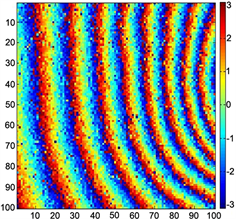Figure 1. Interferogram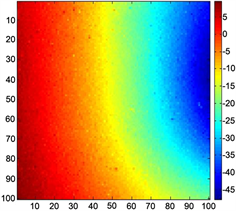Figure 2. Phase derivative variance unwrapped result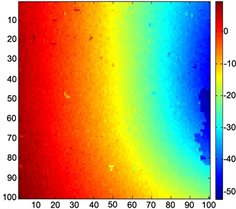Figure 3. Pseudo-coherence coefficient unwrapped resultFigure 4. Maximum phase gradient unwrapped result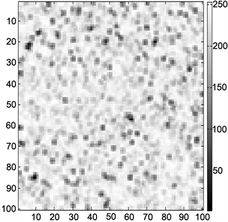(a)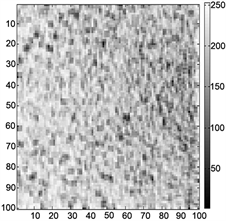(b)(c)

Figure 5. (a) Phase derivate variance map; (b) Pseudo coherence map; (c) Maximum phase gradient map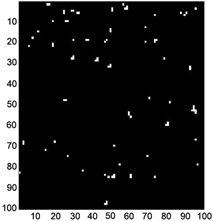(a)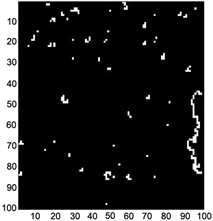(b)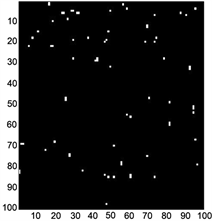(c)

Figure 6. (a) Phase derivative variance discontinuous points map; (b) Pseudo-coherence coefficient discontinuous points map; (c) Maximum phase gradient discontinuous points map

$\epsilon =\frac{1}{MN}\underset{i=0}{\overset{M-2}{\sum }}\underset{j=0}{\overset{N-1}{\sum }}{\omega }_{i,j}^{x}{|{\varphi }_{i+1,j}-{\varphi }_{i,j}-{\Delta }_{i,j}^{x}|}^{p}+\frac{1}{MN}\underset{i=0}{\overset{M-1}{\sum }}\underset{j=0}{\overset{N-2}{\sum }}{\omega }_{i,j}^{y}{|{\varphi }_{i,j+1}-{\varphi }_{i,j}-{\Delta }_{i,j}^{x}|}^{p}$ (8)Table 1. Three quality maps phase unwrapping results’ number of discontinuous points and ε value

4.2. 地形平坦条件下的实验结果与分析Figure 7. InterferogramFigure 8. Phase derivative variance unwrapped result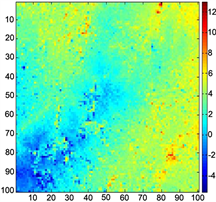Figure 9. Pseudo-coherence coefficient unwrapped result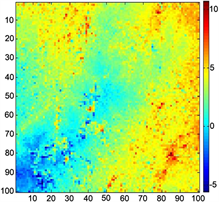Figure 10. Maximum phase gradient unwrapped result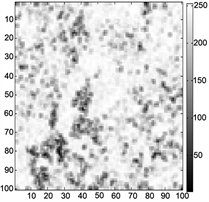(a)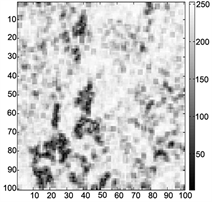(b)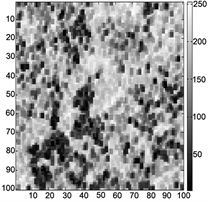(c)

Figure 11. (a) Phase derivate variance map; (b) Pseudo coherence map; (c) Maximum phase gradient map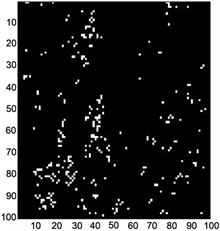(a)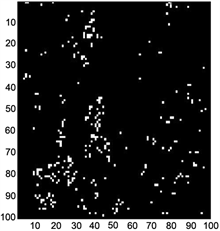(b)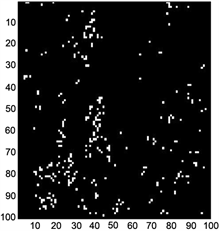(c)

Figure 12. (a) Phase derivative variance discontinuous points map; (b) Pseudo-coherence coefficient discontinuous points map; (c) Maximum phase gradient discontinuous points mapTable 2. Three quality maps phase unwrapping results’ number of discontinuous points and ε value

5. 结论

  Xu, W. and Cumming, I. (1999) A Region-Growing Algorithm for InSAR Phase Unwrapping. IEEE Transactions on Geoscience and Remote Sensing, 37, 124-133. https://doi.org/10.1109/36.739143  Flynn, T.J. (1997) Two Dimensional Phase Unwrapping with Minimum Weighted Discontinuity. Journal of the Optical Society of America, 14, 2692-2701. https://doi.org/10.1364/JOSAA.14.002692  Goldstein, R.M., Zerber, H.A. and Werner, C.L. (1988) Satellite Radar Interferometry: Two-Dimensional Phase Unwrapping. Radio Science, 23, 713-720. https://doi.org/10.1029/RS023i004p00713  Pritt, M.D. and Shipman, L.S. (1994) Least-Squares Two-Dimensional Phase Unwrapping Using FFTs. IEEE Transactions on Geoscience and Remote Sensing, 32, 706-708. https://doi.org/10.1109/36.297989  Pritt, M.D. (1996) Phase Unwrapping by Means of Multigrid Techniques for Interferometric SAR. IEEE Transactions on Geoscience and Remote Sensing, 34, 728-738. https://doi.org/10.1109/36.499752  CHEN, C.W. (2001) Statistical-Cost Network-Flow Approaches to Two-Dimensional Phase Unwrapping for Radar Interferometry. Stanford University, Stanford.  Chen, C.W. (2001) Statistical-Cost Network-Flow Ap-proaches to Two-Dimensional Phase Unwrapping for Radar Interferometry. Stanford University, Stanford, California, USA.  Loffeld, O. and Krämer, R. (1999) Phase Unwrapping for SAR Interferometry—A Data Fusion Approach by Kalman Filtering. IEEE 1999 International Geoscience and Remote Sensing Symposium (IGARSS’99), Hamburg, 28 June-2 July 1999, 1715-1717. https://doi.org/10.1109/IGARSS.1999.772071  Krämer, R. and Loffeld, O. (1996) Phase Unwrapping for SAR In-terferometry with Kalman Filters. EUSAR’96, Königswinter, Germany, 26-28 March 1996, 199-202.  Krämer, R. and Loffeld, O. (1997) Presentation of an Improved Phase Unwrapping Algorithm Based on Kalman Filters Combined with Local Slope Estimation. Fringe 96, Zurich, Suisse, 253-260.  Ghiglia, D.C. and Pritt, M.D. (1998) Two-Dimensional Phase Unwrapping: Theory, Algo-rithms and Software. John Wiley & Sons, Hoboken.